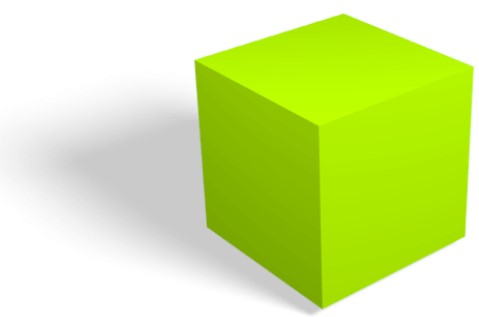# Cube Calculator

Enter the length of side to find the volume, surface area, and diagonal of a cube using cube calculator.

Formula:
Volume = (a3) = (a x a x a)

Give Us Feedback

Cube calculator is an online geometrical tool that takes length of a side and calculates various parameters of a cube including:

• Surface area
• Volume
• Diagonal

## What is a cube?

cube is a three-dimensional solid object bounded by six square faces with three meeting at each vertex. It has 8 vertices, 12 edges, and 6 faces. It is a trigonal trapezohedron in four orientations and regular square prism in three orientations.## Cube formulas

### Volume of cube

Cube volume formula can be written as:

V = a³

### Surface area of cube

Surface area of cube formula can be expressed as:

S = 6 × a²

### Diagonal of cube

The formula for diagonal of cube can be stated as:

D = √3×a

Where,

a is length of side,

V is volume,

S is surface area, and

D is diagonal of cube.

Our cube volume calculator uses all of the above formulas to find these cube parameters.

## How to calculate volume of cube?

Example: If length of side of cube is 8 cm, find the volume of cube.

Cube calc: find V

Step 1: Identify the given values.

a = 8 cm

Step 2: Place the value in volume of cube equation.

V = a³

V = 8³ = 8 × 8 × 8

V = 512 cm3

### How to calculate surface area of cube?

Example: If length of side of cube is 3.5 cm, find the surface area of cube.

Cube calc: find S

Step 1: Identify the given values.

a = 3.5 cm

Step 2: Place the value in surface area of cube equation.

S = 6 × a²

S = 6 × 3.5²

S = 73.5 cm2

### How to find diagonal of cube?

Example: If length of side of cube is 10 cm, find the diagonal length of cube.

Cube calc: find d

Step 1: Identify the given values.

a = 10 cm

Step 2: Place the value in surface area of cube equation.

D = √3×a

D = √3×10

D = 17.32 cm

### Math Tools